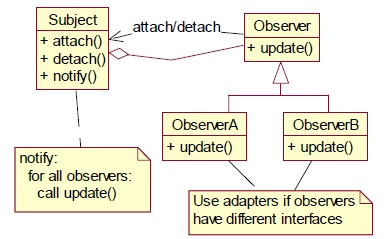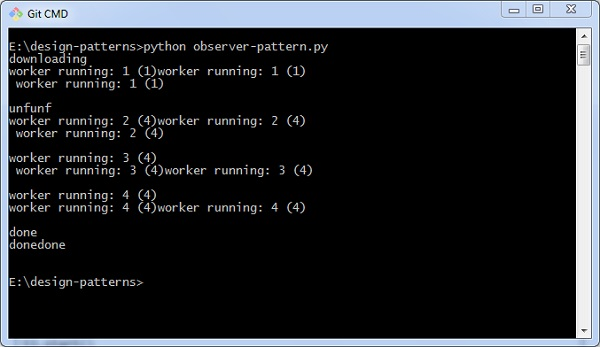# Python Design Patterns - Observer

In this pattern, objects are represented as observers that wait for an event to trigger. An observer attaches to the subject once the specified event occurs. As the event occurs, the subject tells the observers that it has occurred.

The following UML diagram represents the observer pattern −## How to implement the observer pattern?

Let us now see how to implement the observer pattern.

```import threading
import time
import pdb

def run(self):
for i in range(1,5):
self.i = i
time.sleep(2)
print 'unfunf'
return 'hello world'

def run(self):
for i in range(1,5):
print 'worker running: %i (%i)' % (i, t.i)
time.sleep(1)
t.join()

print 'done'

t.start()

time.sleep(1)

t1 = Worker()
t1.start()

t2 = Worker()
t2.start()

t3 = Worker()
t3.start()
```

### Output

The above program generates the following output −### Explanation

The above code explains the procedure of downloading a particular result. As per the observer pattern logic, every object is treated as observer. It prints the output when event is triggered.